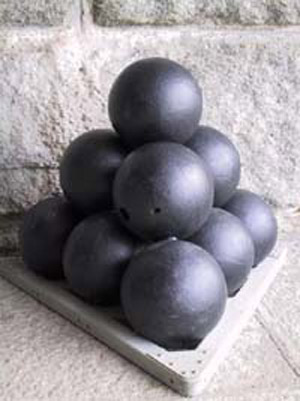A# cannonball problemA tetrahedral stack of cannonballs.

The mathematical analysis of stacks of cannonballs – and of spheres in general – has its roots in a question posed by Sir Walter Raleigh, favorite of Queen Elizabeth I, explorer, introducer of the potato and tobacco to Britain, and part-time pirate on the high seas. Raleigh asked his mathematical assistant, Thomas Harriot, how he could quickly figure out the number of cannonballs in a square pyramidal stack (the accompanying photo is actually of a tetrahedral stack). In other words, given how many cannonballs ran along the side of the bottom layer, how could the total number of cannonballs in the stack be figured out without having to count them individually. Harriot solved this problem without difficulty. If k is the number of cannonballs along the side of the bottom layer, the number of cannonballs in the pyramid, n, is equal to 1/6 k(1 + k)(1 + 2k). For example, if k = 7, n = 140.

A more specific form of the cannonball problem asks what is the smallest number of balls that can first be laid out on the ground as an n × n square, then piled into a square pyramid k balls high? In other words, what is the smallest square number that is also square pyramidal number? This answer is the smallest solution to the Diophantine equation

1/6 k(1 + k)(1 + 2k) = n2

and turns out to be k = 24, n = 70, corresponding to 4,900 cannonballs. The ultimate form of the cannonball problem is to ask if there are any other, larger solutions. In 1875, Edouard Lucas conjectured that there weren't and in 1918 G. N. Watson proved that Lucas was right.1

Returning to Elizabethan times, Thomas Harriot's interest in spheres extended far beyond piles of cannonballs. Harriot was an atomist (see atomism), in the classical Greek sense, and believed that understanding how spheres pack together was crucial to understanding how the basic constituents of nature are arranged. Harriot also carried out numerous experiments in optics and was far ahead of his time in this field. So, when, in 1609, Johannes Kepler wanted some advice on how to give his own theories on optics a stronger scientific underpinning who better to turn to than the Englishman? Harriot supplied Kepler with important data on the behavior of light rays passing through glass, but he also stimulated the German's interest in the sphere-packing problem. In response, Kepler published a little booklet titled The Six-Cornered Snowflake (1611) that would influence the science of crystallography for the next two centuries and that contained what has come to be known as Kepler's conjecture about the most efficient way to pack spheres.

### Reference

1. Watson, G. N. "The Problem of the Square Pyramid." Messenger of Mathematics, 48: 1-22 (1918-1919).